# TypeError: can only concatenate tuple (not "X") to tupleLast updated: Jan 30, 2023
6 min## #TypeError: can only concatenate tuple (not "int") to tuple

The Python "TypeError: can only concatenate tuple (not "int") to tuple" occurs when we try to concatenate a tuple and an integer.

To solve the error, make sure you haven't got any dangling commas if trying to sum two integers, otherwise declare 2 tuples.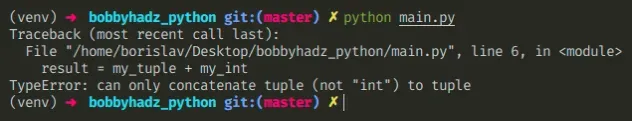Here is an example of how the error occurs.

main.py
```Copied!```my_tuple = 1, 2, 3

my_int = 4

# ⛔️ TypeError: can only concatenate tuple (not "int") to tuple
result = my_tuple + my_int
``````

Trying to use the addition (+) operator with a tuple and an integer causes the error.

The error also occurs when working with floating-point numbers.

main.py
```Copied!```my_tuple = 1.1, 2.2

my_float = 3.3

# ⛔️ TypeError: can only concatenate tuple (not "float") to tuple
result = my_tuple + my_float
``````

We tried to use the addition (+) operator to concatenate a tuple and an integer which caused the error.

The values on the left and right-hand sides need to be of compatible types.

## #Concatenating two tuples

If you meant to concatenate two tuples, declare a tuple instead of an int.

main.py
```Copied!```my_tuple = 1, 2, 3

my_other_tuple = (4,)

result = my_tuple + my_other_tuple

print(result)  # 👉️ (1, 2, 3, 4)
``````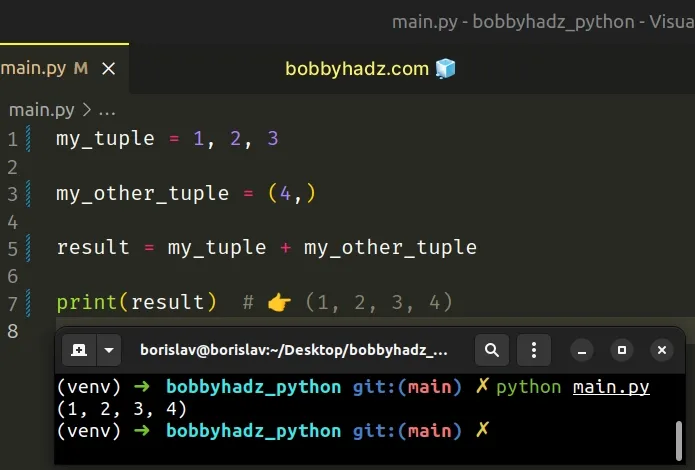When you use the addition (+) operator with two tuples, the elements of the tuples get combined into a new tuple.

If you meant to add 2 integers, make sure you don't have any dangling commas as you might be declaring a tuple by mistake.
main.py
```Copied!```my_tuple = 4, # 👈️ comma makes it a tuple

print(type(my_tuple)) # 👉️ <class 'tuple'>
``````

Having a trailing comma after the integer declares a tuple.

You can remove the trailing comma to declare an integer.

main.py
```Copied!```int_1 = 4
int_2 = 6

result = int_1 + int_2
print(result) # 👉️ 10
``````

If you meant to declare two integers on the same line, use unpacking.

main.py
```Copied!```int_1, int_2 = 4, 10

print(int_1)  # 👉️ 4
print(int_2)  # 👉️ 10
``````

Note that the values on the left have to be exactly as many as the values on the right of the equal sign.

## #How tuples are constructed in Python

Tuples are constructed in multiple ways:

• Using a pair of parentheses `()` creates an empty tuple
• Using a trailing comma - `a,` or `(a,)`
• Separating items with commas - `a, b` or `(a, b)`
• Using the `tuple()` constructor

## #Accessing a tuple element at an index

If you meant to access an element at a specific index in the tuple, use square brackets.

main.py
```Copied!```my_tuple = 1, 2, 3

my_int = 10

result = my_tuple + my_int

print(result)  # 👉️ 11
``````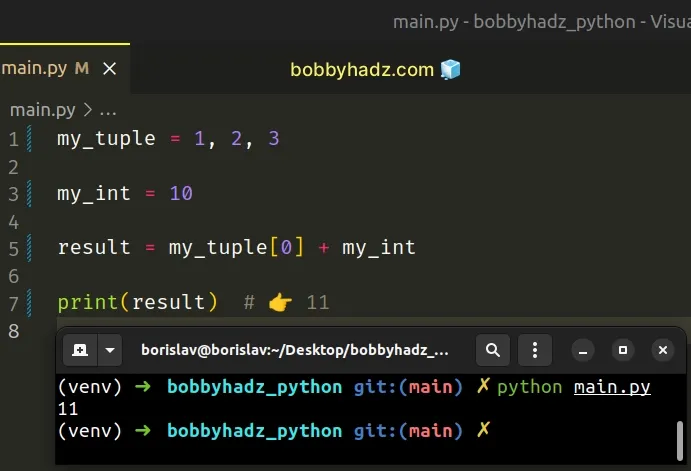We accessed the first element in the tuple and added it to an integer.

Note that the values on both sides of the addition operator are integers, so this is allowed.

Python indexes are zero-based, so the first item in a tuple has an index of `0`, and the last item has an index of `-1` or `len(my_tuple) - 1`.

## #Checking the type of a variable

If you aren't sure what type a variable stores, use the built-in `type()` class.

main.py
```Copied!```my_tuple = (1, 2, 3)
print(type(my_tuple))  # 👉️ <class 'tuple'>
print(isinstance(my_tuple, tuple))  # 👉️ True

my_int = 10
print(type(my_int))  # 👉️ <class 'int'>
print(isinstance(my_int, int))  # 👉️ True
``````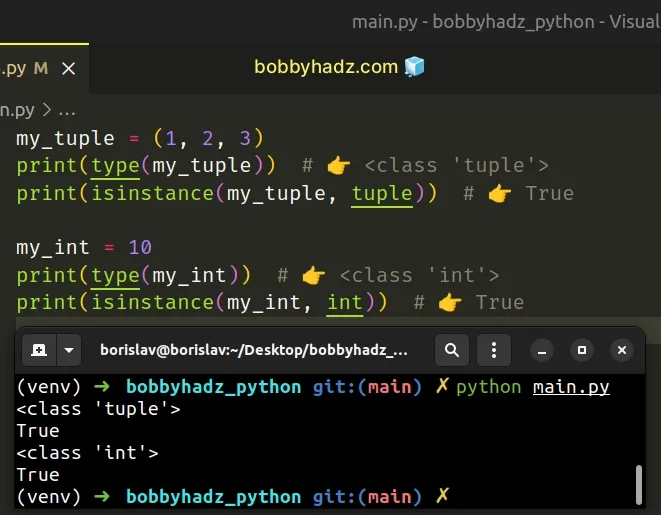The type class returns the type of an object.

The isinstance function returns `True` if the passed-in object is an instance or a subclass of the passed-in class.

## #TypeError: can only concatenate tuple (not "str") to tuple

The Python "TypeError: can only concatenate tuple (not "str") to tuple" occurs when we try to concatenate a tuple and a string.

To solve the error, make sure that the values are either 2 tuples or 2 strings before concatenating them.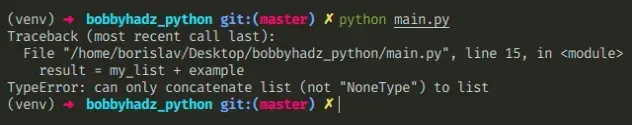Here is an example of how the error occurs.

main.py
```Copied!```my_tuple = ('apple', 'banana')

my_str = 'kiwi'

# ⛔️ TypeError: can only concatenate tuple (not "str") to tuple
result = my_tuple + my_str
``````

We tried to use the addition (+) operator to concatenate a tuple and string which caused the error.

The values on the left and right-hand sides need to be of compatible types.

## #Concatenating two tuples

If you meant to concatenate two tuples, declare a tuple instead of a string.

main.py
```Copied!```my_tuple = ('apple', 'banana')

my_other_tuple = ('kiwi',)

result = my_tuple + my_other_tuple

print(result)  # 👉️ ('apple', 'banana', 'kiwi')
``````

If you meant to concatenate 2 strings, make sure the two variables store a string.

When you declare a variable with a trailing comma, you declare a tuple.

main.py
```Copied!```my_tuple = 'apple', # 👈️ note the trailing comma

print(my_tuple) # 👉️ ('apple',)
print(type(my_tuple)) # 👉️ <class 'tuple'>
``````

If you meant to declare a string, remove the trailing comma.

If you meant to declare two strings on the same line, use unpacking.

main.py
```Copied!```str_1, str_2 = ('one', 'two')

print(str_1) # 👉️ one
print(str_2) # 👉️ two
``````

## #How tuples are created in Python

Tuples are constructed in multiple ways:

• Using a pair of parentheses `()` creates an empty tuple
• Using a trailing comma - `a,` or `(a,)`
• Separating items with commas - `a, b` or `(a, b)`
• Using the `tuple()` constructor

## #Accessing a specific element ina tuple.

If you meant to access an element at a specific index in the tuple, use square brackets.

main.py
```Copied!```my_tuple = ('apple', 'banana')

my_str = ' is a fruit'

result = my_tuple + my_str

print(result)  # 👉️ "apple is a fruit"
``````

We concatenated the first element in the tuple to a string. Note that the values on both sides of the addition (+) operator are strings, so this is allowed.

Python indexes are zero-based, so the first item in a tuple has an index of `0`, and the last item has an index of `-1` or `len(my_tuple) - 1`.

## #Printing the contents of a tuple

If you want to print the contents of a tuple, use a formatted string literal.

main.py
```Copied!```my_tuple = ('apple', 'banana')

my_str = 'are fruits'

result = f'{my_tuple} {my_str}'

print(result)  # 👉️ "('apple', 'banana') are fruits"
``````
Formatted string literals (f-strings) let us include expressions inside of a string by prefixing the string with `f`.

Make sure to wrap expressions in curly braces - `{expression}`.

## #Checking the type of a variable

If you aren't sure what type a variable stores, use the built-in `type()` class.

main.py
```Copied!```my_tuple = ('apple', 'banana')
print(type(my_tuple))  # 👉️ <class 'tuple'>
print(isinstance(my_tuple, tuple))  # 👉️ True

my_str = 'are fruits'
print(type(my_str))  # 👉️ <class 'str'>
print(isinstance(my_str, str))  # 👉️ True
``````

The type class returns the type of an object.

The isinstance function returns `True` if the passed-in object is an instance or a subclass of the passed-in class.

## #TypeError: can only concatenate tuple (not "list") to tuple

The Python "TypeError: can only concatenate tuple (not "list") to tuple" occurs when we try to concatenate a tuple and a list.

To solve the error, make sure the two values are of type list or type tuple before concatenating them.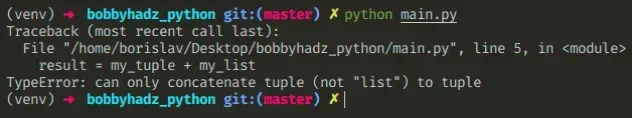Here is an example of how the error occurs.

main.py
```Copied!```my_tuple = ('a', 'b')
my_list = ['c', 'd']

# ⛔️ TypeError: can only concatenate tuple (not "list") to tuple
result = my_tuple + my_list
``````

We tried to use the addition (+) operator to concatenate a tuple and a list which caused the error.

The values on the left and right-hand sides need to be of compatible types.

## #Converting the list to a tuple to concatenate two tuples

If you meant to concatenate two tuples, declare a tuple instead of a list, or convert the list to a tuple.

main.py
```Copied!```my_tuple = ('a', 'b')
my_list = ['c', 'd']

result = my_tuple + tuple(my_list)

print(result)  # 👉️ ('a', 'b', 'c', 'd')
``````

The tuple class takes an iterable and returns a `tuple` object.

We converted the list to a tuple and concatenated the two tuples.

## #Converting the tuple to a list to concatenate two lists

Conversely, you can convert the tuple into a list and concatenate the two lists.

main.py
```Copied!```my_tuple = ('a', 'b')
my_list = ['c', 'd']

result = list(my_tuple) + my_list

print(result)  # 👉️ ['a', 'b', 'c', 'd']
``````

The list class takes an iterable and returns a list object.

## #How tuples are created in Python

If you declared the tuple by mistake, tuples are constructed in the following ways:

• Using a pair of parentheses `()` creates an empty tuple
• Using a trailing comma - `a,` or `(a,)`
• Separating items with commas - `a, b` or `(a, b)`
• Using the `tuple()` constructor

If you meant to append the tuple to a list, use the `append()` method.

main.py
```Copied!```my_tuple = ('c', 'd')
my_list = [('a', 'b')]

my_list.append(my_tuple)
print(my_list)  # 👉️ [('a', 'b'), ('c', 'd')]
``````

The list.append() method adds an item to the end of the list.

The method returns `None` as it mutates the original list.

## #Checking what type a variable stores

If you aren't sure what type a variable stores, use the built-in `type()` class.

main.py
```Copied!```my_tuple = ('a', 'b')
print(type(my_tuple))  # 👉️ <class 'tuple'>
print(isinstance(my_tuple, tuple))  # 👉️ True

my_list = ['c', 'd']
print(type(my_list))  # 👉️ <class 'list'>
print(isinstance(my_list, list))  # 👉️ True
``````

The type class returns the type of an object.

The isinstance function returns `True` if the passed-in object is an instance or a subclass of the passed-in class.

I wrote a book in which I share everything I know about how to become a better, more efficient programmer.You can use the search field on my Home Page to filter through all of my articles.